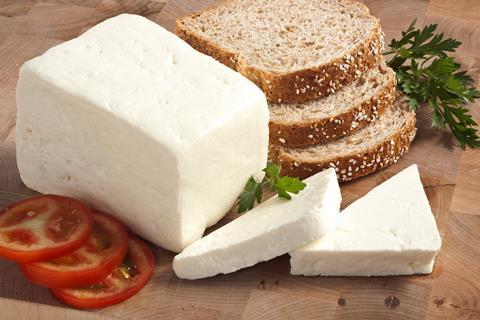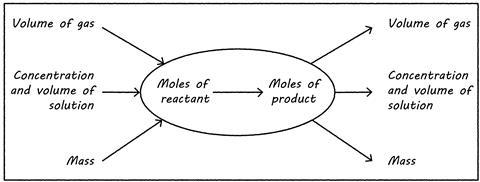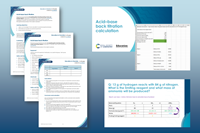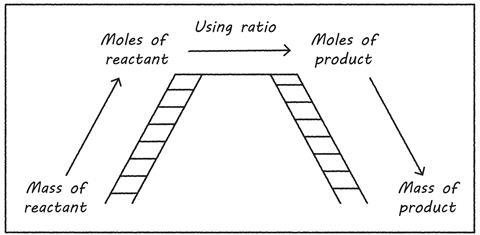Use these classroom tips to effectively teach your post-16 students how reagents react together in the correct proportionsMake some cheese and tomato sandwiches to help your post-16 students understand ratios – and the learning rewards will be tangible (and tasty)

Every reaction in chemistry, be it pharmaceutical synthesis or setting off fireworks, depends on reagents reacting in the right proportions. If we consider a simple reaction, heating sodium metal and placing it in chlorine gas to make sodium chloride, why do we care about reacting masses? Well, sodium chloride is often added to food. If we had excess sodium present, that’s pretty reactive. It would be rather dangerous to accidently put lumps of sodium, which reacts highly exothermically with water, on your cabbage, or have excess chlorine gas, which causes damage to the respiratory system. We must be able to work out exactly how much of each reagent we need. Likewise, it’s important that we work out exactly how much of a product we’ll make.

## What students need to know

Before teaching this concept, you must be sure your students have a good grasp of moles and how they’re used to measure amounts of chemicals. Learners also need to be able to balance equations and understand that the stochiometric numbers in these equations relate to the ratio in which reagents react.

At post-16, many students will have met these ideas before, but will need solidification. You will need to work through some important steps:

1. What a balanced equation tells us.
2. How to convert masses, volumes and concentrations into moles.
3. How to relate the ratio in the balanced equation to work out reacting masses, as well as which reagents are in excess and which are limiting.
4. Converting an amount in moles back into a mass or volume.

It’s especially challenging to link all these steps together in a range of ways, depending on the information you have and what you need to determine (see image).Source: Supplied by author

We can use information to determine moles, and then convert a number of moles into mass, volume of a gas or concentration and volume of a solution

## Common misconceptions

Misconceptions often stem from a lack of understanding of the mole, how to use it in calculations and how to identify the limiting reagent. Linking moles to the ratios present in balanced equations can be confusing. The big numbers tell us the ratio between the substances and the small numbers tell us what a compound is. To support students here, draw out 5H2O to show five H2O molecules.

•Acid–base back titration, for age range 16–18

Use the experiment and accompanying resources to develop your learners' practical and quantitative chemistry skills.

Resources include:

Acid–base back titration, for age range 16–18

Use the experiment and accompanying resources with your learners to develop their quantitative chemistry skills, from the Education in Chemistry  website: rsc.li/3nQJNCi

The most significant challenge for some students will be the mathematical content of this topic. Although what they need to do mathematically is not actually challenging (even if they are not taking maths post-16), a fear of the maths can be a significant barrier for some learners. Scaffolding can be very helpful to ensure students understand what the numbers mean at each stage.

Not all students will conceptualise this topic in the same way. Some will be comfortable with a numerical explanation; others will find models much more useful.Source: Supplied by author

Use this image to bridge the gap for students struggling to understand reacting masses calculations

Approaching it mathematically, you can use the idea of moles as a bridge to support learning (see image). Initially students must be happy converting masses or volumes into moles (the Quantitative chemistry starter for 10 gives lots of practice of this), and using ratios. You can also support students with these ideas on how to apply maths skills in chemistry

Approaching it mathematically, we can use the idea of moles as a bridge to support learning (see image). Initially students must be happy converting masses/volumes into moles – the Quantitative chemistry starter for 10 gives lots of practice of this (rsc.li/3In63KS) – and using ratios. You can also support students with these ideas on how to apply maths skills in chemistry: rsc.li/3MaNr1Y.

For students who find a physical model more useful, ratios are used a lot in cooking. When making sandwiches, you need bread, cheese and tomatoes. If every sandwich has two slices of bread, three tomatoes and one slice of cheese, we can write an equation to show the ratios involved:

2B + 3T + C → S

Physical props like this can really help students – bread, cheese and tomato cut out of different coloured paper – allowing them to ‘make sandwiches’. Provide questions with any amounts of these ingredients, asking them to work out how many sandwiches they can make, what’s the limiting reagent (ie what stops them being able to make more sandwiches) and what’s left over. For example, if I have six slices of bread, eight slices of tomato and six slices of cheese, how many sandwiches can I make and what’s the limiting ingredient? When students understand the idea of a limiting reagent in this easy-to-conceptualise example, it’s easier to transfer knowledge to the more abstract example of chemicals.

## More resources

It’s also important to ensure that students have a clear method for writing out their calculation steps, as confusion can easily lead to the wrong answer here, even if the student understands what’s going on. Scaffolding this can help them remain clear at each step. Download the accompanying PowerPoint slides (also available as pdf) showing how to find the limiting reagent and mass of ammonia produced when 13 g of hydrogen reacts with 84 g of nitrogen.

Students sometimes find it challenging to calculate the limiting reagent, as they need to know the number of moles for each substance, as well as the ratio needed. So, it’s important to clearly explain that the limiting reagent may not be the one they have the least number of moles of.

## Checking for understanding

You can use questions from evidence-based research to check conceptual understanding of reacting masses and limiting reagents. Students should work in small groups, discussing each step and correcting each other as they go. Encourage students to explain why they carry out each step to ensure understanding. Make sure you check all the working as well as the final answer.

You can use questions from evidence-based research (bit.ly/3MC67ZP) to check conceptual understanding of reacting masses and limiting reagents. Students should work in small groups, discussing each step and correcting each other as they go. Encourage students to explain why they carry out each step to ensure understanding. Make sure you check all the working as well as the final answer.

## Take-home points

To allow students to confidently complete reacting mass and limiting reagent questions, build their understanding in steps:

1. Ensure they understand the mole concept.
2. Make sure they understand the ratios in balanced equations.
3. Support them in building an understanding of how these link together to work out the amounts of each reactant, and what’s left over – both mathematically and conceptually.

Article and resource by Jo Haywood, who teaches chemistry at secondary school and undergraduate level and leads chemistry on the University of Cambridge PGCE course. Technician notes adapted by Sandrine Bouchelkia